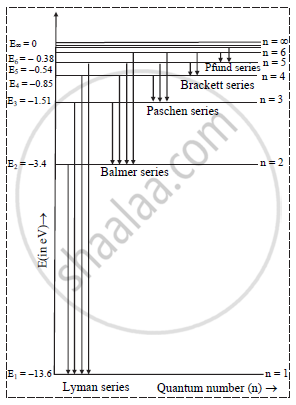HSC Science (General) 12th Board ExamMaharashtra State Board
Share

Books Shortlist

# Draw a Neat, Labelled Energy Level Diagram for H Atom Showing the Transitions. Explain the Series of Spectral Lines for H Atom, Whose Fixed Inner Orbit Numbers Are 3 and 4 Respectively. - HSC Science (General) 12th Board Exam - Physics

#### Question

Draw a neat, labelled energy level diagram for H atom showing the transitions. Explain the series of spectral lines for H atom, whose fixed inner orbit numbers are 3 and 4 respectively.

#### SolutionPaschen series:
i. The spectral lines of this series correspond to the transition of an electron from some higher energy state to 3rd orbit.
ii. For paschen series, p = 3 and n = 4, 5,...
The wave numbers and the wavelengths of the spectral lines constituting the Paschen series are given by,

barv=1/lambda=R(1/3^2-1/n^2)

iii. Paschen series lies in the infrared region of the spectrum which is invisible and contains infinite number of lines.

iv. Wavelengths for n = 4 and 5 are 18750 Å and 12820 Å respectively.

Brackett series:
i. The spectral lines of this series corresponds to the transition of an electron from a higher energy state to the 4th orbit.
ii. For this series, p = 4 and n = 5, 6, 7,...
The wave numbers and the wavelengths of the spectral lines constituting the Brackett series are given by,

barv=1/lambda=R(1/4^2-1/n^2)

iii. This series lies in the ne ar infrared region of the spectrum and contains infinite number of lines. Wavelengths for n = 5 and 6, are 40518 Å and 26253 Å respectively

Is there an error in this question or solution?

#### APPEARS IN

2016-2017 (March) (with solutions)
Question 8.2 | 7.00 marks

#### Video TutorialsVIEW ALL 

Solution Draw a Neat, Labelled Energy Level Diagram for H Atom Showing the Transitions. Explain the Series of Spectral Lines for H Atom, Whose Fixed Inner Orbit Numbers Are 3 and 4 Respectively. Concept: Bohr'S Model for Hydrogen Atom.
S